## Example Questions

### Example Question #1 : How To Add Odd Numbers

Which of the following could represent the sum of 3 consecutive odd integers, given that d is one of the three?

3d + 3

3d – 9

3d – 3

3d – 6

3d + 4

3d – 6

Explanation:

If the largest of the three consecutive odd integers is d, then the three numbers are (in descending order):

dd – 2, d – 4

This is true because consecutive odd integers always differ by two. Adding the three expressions together, we see that the sum is 3d – 6.

### Example Question #2 : How To Add Odd Numbers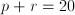, where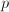andare distinct positive integers.  Which of the following could be values ofand?

0 and 20

–10 and 30

4 and 5

5 and 15

10 and 10

5 and 15

Explanation:

Sinceandmust be positive, eliminate choices with negative numbers or zero. Since they must be distinct (different), eliminate choices where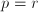.  This leaves 4 and 5 (which is the only choice that does not add to 20), and the correct answer, 5 and 15.

### Example Question #1 : Integers

The sum of three consecutive odd integers is 93. What is the largest of the integers?Explanation:

Consecutive odd integers differ by 2. If the smallest integer is x, then

x + (x + 2) + (x + 4) = 93

3x + 6 = 93

3x = 87

x = 29

The three numbers are 29, 31, and 33, the largest of which is 33.

### Example Question #8 : How To Add Odd Numbers

Solve: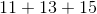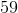Explanation: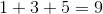Since there is no tens digit to carry over, proceed to add the tens digits: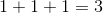The answer is.

### Example Question #9 : How To Add Odd Numbers

At a certain high school, everyone must take either Latin or Greek. There aremore students taking Latin than there are students taking Greek. If there are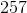students taking Greek, how many total students are there?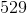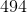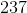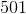Explanation:

If there arestudents taking Greek, then there are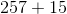or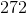students taking Latin. However, the question asks how many total students there are in the school, so you must add these two values together to get: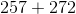ortotal students.

### Example Question #1 : Integers

Add: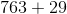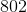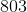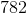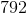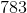Explanation: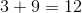Since the there is a tens digit, use that as the carryover to the next term.

Add the tens digit including the carryover.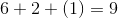The hundreds digit is 7.

Combine the ones digit of each calculation in order.

The answer is:### Example Question #2 : Integers

Add: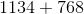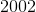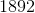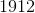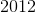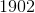Explanation: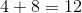Carry over the one from the tens digit to the next number.

Add the tens digit with the carry over.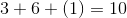Carry over the one from the tens digit to the hundreds digit.

Add the hundreds digit with the carry over.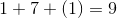The thousands digit has no carry over.  The second number has no thousands digit.  This means that the thousands is one.  Combine all the ones digits from each of the previous calculations.

The correct answer is: